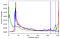# Unveiling Meaning: Using Python for Image Enhancements (White Balancing)

We’re living in a world overrun by filters and airbrushed models. However, I personally believe that beauty and meaning are found where truth is. The same goes for images. So, we perform image enhancements, primarily to restore images to how they should really look.

This article will discuss the following white balancing techniques for image enhancement:

• White Patch Algorithm

For the white balancing algorithms, we will be using this photo:

Before anything, let us load the image.

`import numpy as npimport skimage.io as skiofrom skimage import img_as_ubyte, img_as_floatimg1 = skio.imread('img1.jpg')`

## White Patch Algorithm

The white patch algorithm presumes that the true white of an image occurs at a level lower than the current white levels (R 255, G 255, B 255) of each channel. Thus we will rescale each channel’s values so that everything below the threshold of the true white will be stretched towards 255, while everything above this threshold will be set to 255. To visualize these values for our image, let us graph the proportion of pixels for each channel value relative to the total number of pixels for the channel.

`for channel, color in enumerate('rgb'):    print(channel)    channel_values = img1[:,:,channel]    plt.step(np.arange(256),              np.bincount(channel_values.flatten(), minlength=256)*1.0/                         channel_values.size,              c=color)    plt.xlim(0, 255)    plt.axvline(np.percentile(channel_values, 95), ls='--', c=color)plt.xlabel('channel value')plt.ylabel('fraction of pixels');`Figure 1. Fraction of pixels for each channel value out the total number of pixels per channel

In Figure 1, we also marked the channel value of the 95th percentile for each channel.

`for channel, color in enumerate('RGB'):    print('95th percentile of %s channel:'%channel,           np.percentile(img1[:,:,channel], 95))`

We then obtain these values for the 95th percentile of each RGB channel:

• R Channel: 253.0

We then rescale the channel values such that the above values consist of white pixels.

`img1_wp = img_as_ubyte((img1*1.0 / np.percentile(img1, 95, axis=(0, 1))).clip(0, 1))skio.imshow(img1_wp)`

We then obtain the following white-balanced image using the white patch algorithm:

With such algorithm, we have decreased the sepia-like tint over the image. Let’s see if the other algorithms will perform as well or better.

## Gray-World Algorithm

The gray-world algorithm is similar to the white patch algorithm in that it rescales all the values based on a specific value per channel. In this instance we use the mean value of the image and rescale each channels values such that all channels will have the same mean as that of the image. This is based on the assumption that on average (or if we combine all pixels) we end up with a gray color. This rationale of a gray-world might not be so intuitive, however, if you the reader will get the chance, try mixing red, green, and blue paints and you will end up with a gray color.

`img1_gw = ((img1 * (img1.mean() / img1.mean(axis=(0, 1))))             .clip(0, 255).astype(int))skio.imshow(img1_gw);`

It seems for the image we want to enhance, the gray-world algorithm has little to no effect. This only suggests that the mean value for each channel is already close, if not equal, to the mean value of the entire image. And for our last white-balancing technique, we apply the ground-truth algorithm.

## Ground-Truth Algorithm

The ground-truth algorithm is similar to the white patch algorithm in that it rescales the image based on a true white value. However, instead of setting a threshold based on the channel values, we manually choose a region of the image which we believe represents the true white.

`from matplotlib.patches import Rectanglefig, ax = plt.subplots()ax.imshow(img1)ax.add_patch(Rectangle((340, 50), 20, 20, edgecolor='r', facecolor='none'));`

Let’s zoom in to this true white region:

`img1_patch = img1[50:70, 340:360]skio.imshow(img1_patch);`

There are two ways to use this selected region: we can either rescale the values based on (i) the maximum values of the RGB channels of the region as the threshold for the white values of the image, or (ii) the mean values of the RGB channels of the image matched to the mean values of the same for the patch.

With the first option, we obtain:

`img1_gt_max = (img1*1.0 / img1_patch.max(axis=(0, 1))).clip(0, 1)skio.imshow(img1_gt_max);`

Unfortunately, the change is only mostly noticeable on the brighter areas of the image such that these whites are more pronounced. However, the tint generally still remains. For the second option in using the mean values:

`img1_gt_mean = ((img1 * (img1_patch.mean() / img1.mean(axis=(0, 1)))).clip(0, 255).astype(int))skio.imshow(img1_gt_mean);`

Using the mean produced the worst result among all the algorithms. We can infer that this is because the mean values of the patch are higher than the mean values of the image, thus skewing too much of the colors to a higher brightness. However, this method might work for brighter images as most values will be closer to the true white patch.

In this article, we were able to perform image enhancements using various white balancing techniques. As we have seen, each algorithm outputs various results. While one algorithm might work better for one image, another image might need a different algorithm. We can associate this to the fact that images have different characteristics from one another, including those that need enhancements.

Please visit my blog post on Part 2 of Image Enhancements where we perform histogram manipulation.

Am I doing this data science thing right?

## More from Tonee Bayhon

Am I doing this data science thing right?